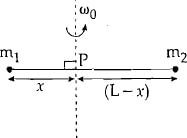#### Point masses m1 and m2 are placed at the opposite ends of a rigid rod of length L, and negligible mass. The rod is to be set rotating about an axis perpendicular to it. The position of point P on this rod through which the axis should pass so that the work required to set the rod rotating with angular velocityis minimum, is given by :Option 1)Option 2)Option 3)Option 4)As we learned in concept

Moment of inertia for system of particle -- wherein

Applied when masses are placed discretely.

Total M.I of rodAs I is minimum i.e.=>Option 1)this is the incorrect option

Option 2)this is the incorrect option

Option 3)this is the correct option

Option 4)this is the incorrect option

#### Plabita